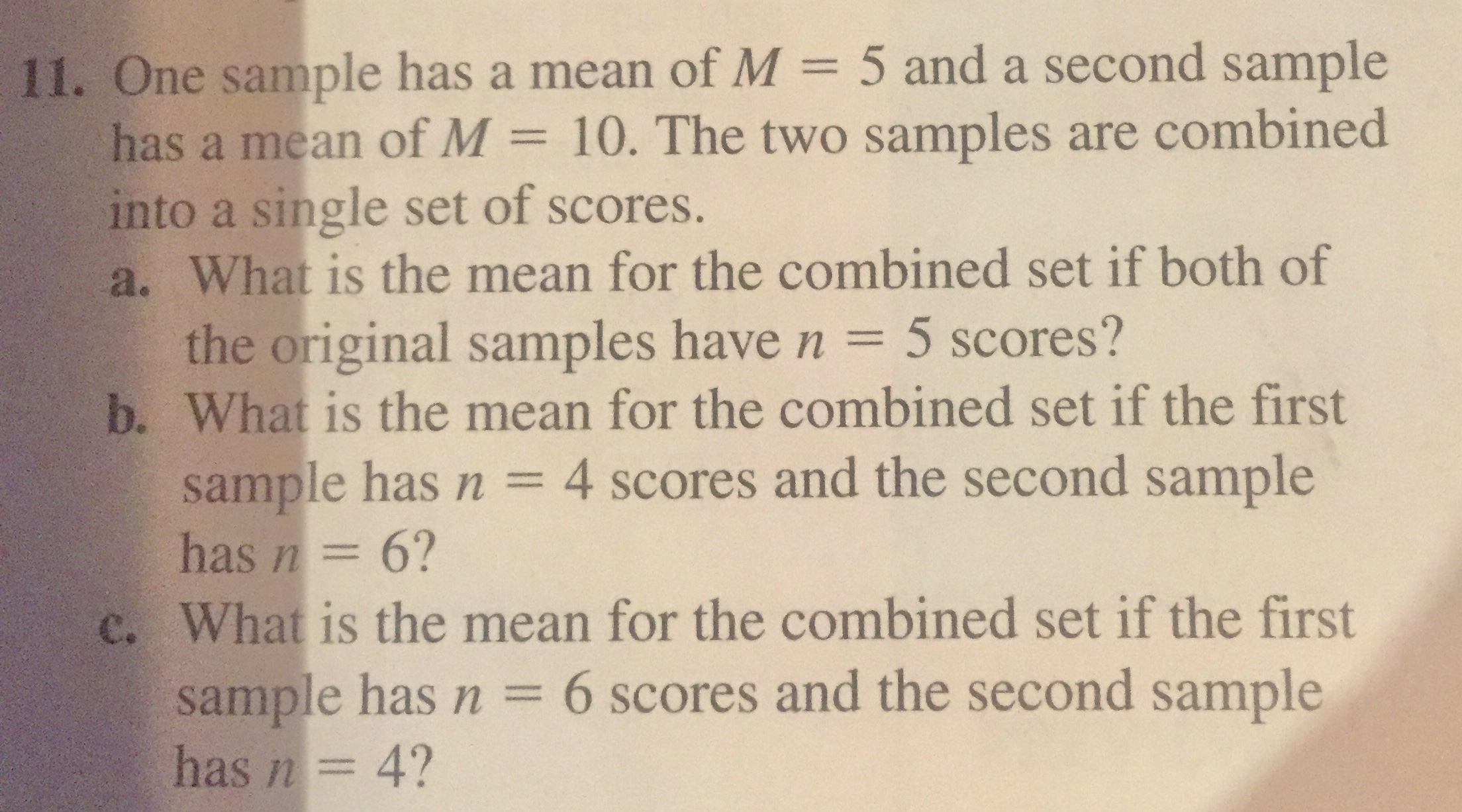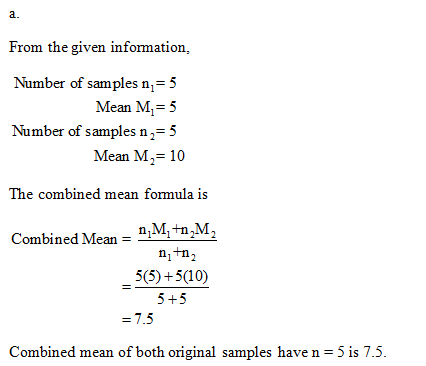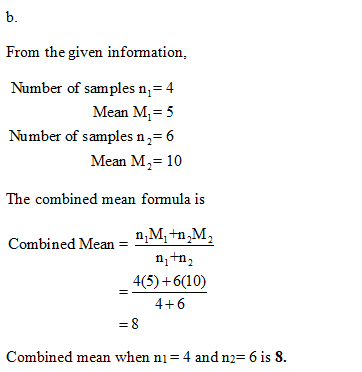# 11. One sample has a mean of M = 5 and a second samplehas a mean of M = 10. The two samples are combinedinto a single set of scores.a. What is the mean for the combined set if both ofthe original samples have n = 5 scores?b. What is the mean for the combined set if the firstsample has n = 4 scores and the second samplehas n= 6?c. What is the mean for the combined set if the firstsample has n = 6 scores and the second samplehas n= 4?%3D

Question
14 viewshelp_outlineImage Transcriptionclose11. One sample has a mean of M = 5 and a second sample has a mean of M = 10. The two samples are combined into a single set of scores. a. What is the mean for the combined set if both of the original samples have n = 5 scores? b. What is the mean for the combined set if the first sample has n = 4 scores and the second sample has n= 6? c. What is the mean for the combined set if the first sample has n = 6 scores and the second sample has n= 4? %3D fullscreen
check_circle

Step 1Step 2...

### Want to see the full answer?

See Solution

#### Want to see this answer and more?

Solutions are written by subject experts who are available 24/7. Questions are typically answered within 1 hour.*

See Solution
*Response times may vary by subject and question.
Tagged in